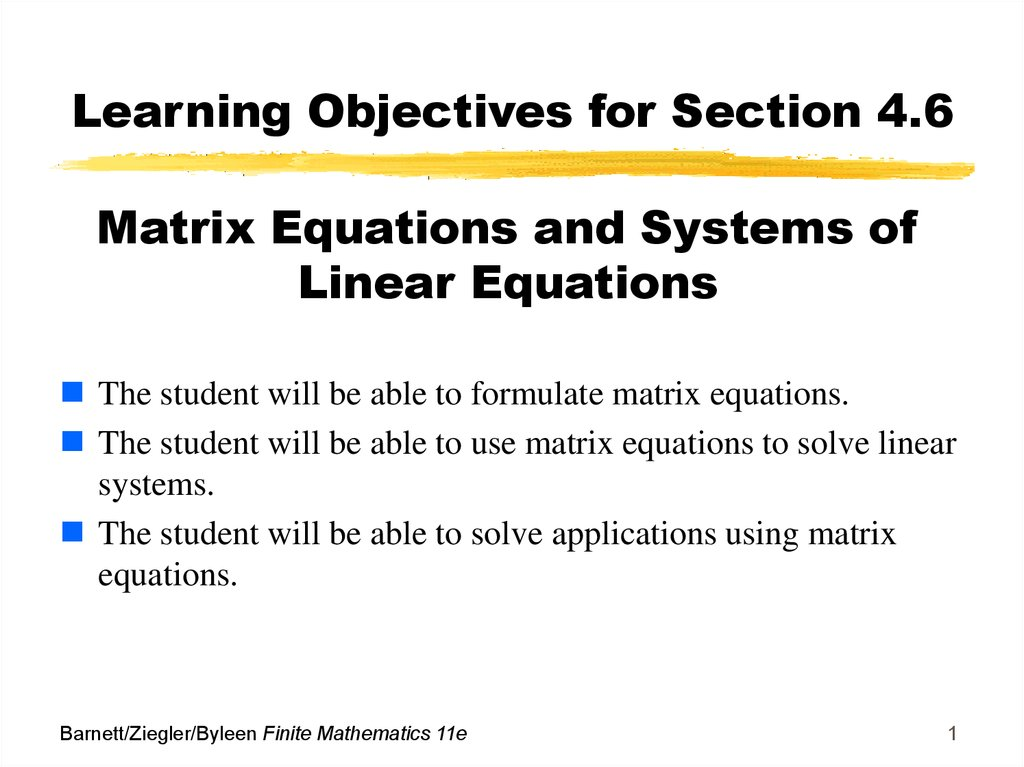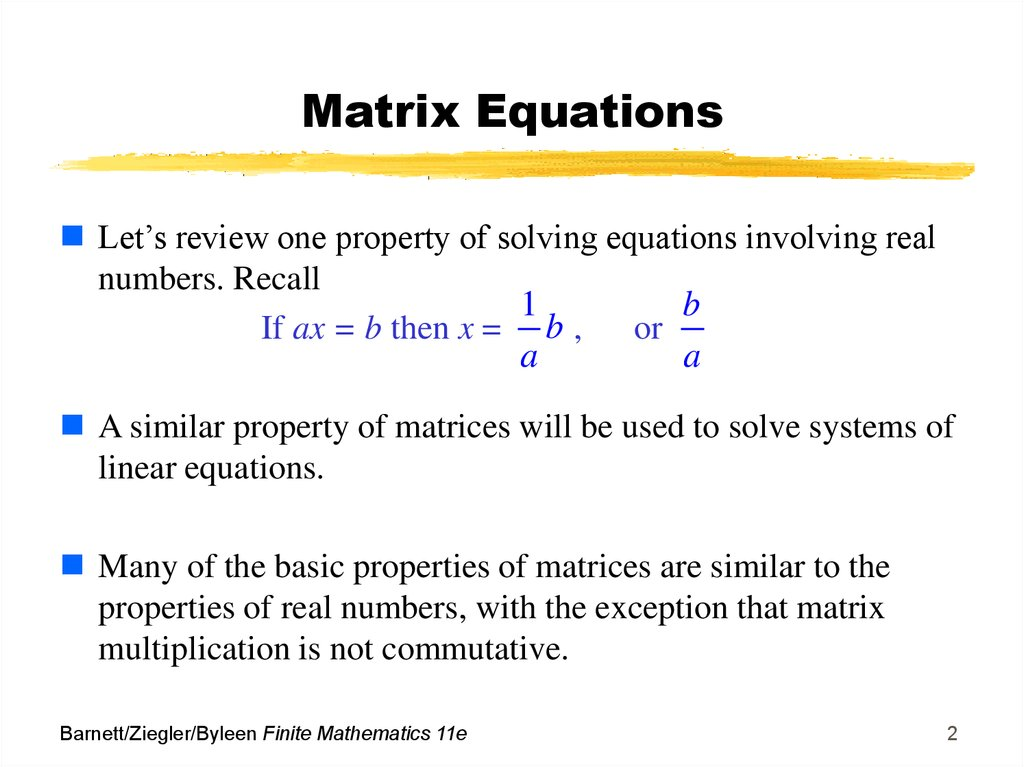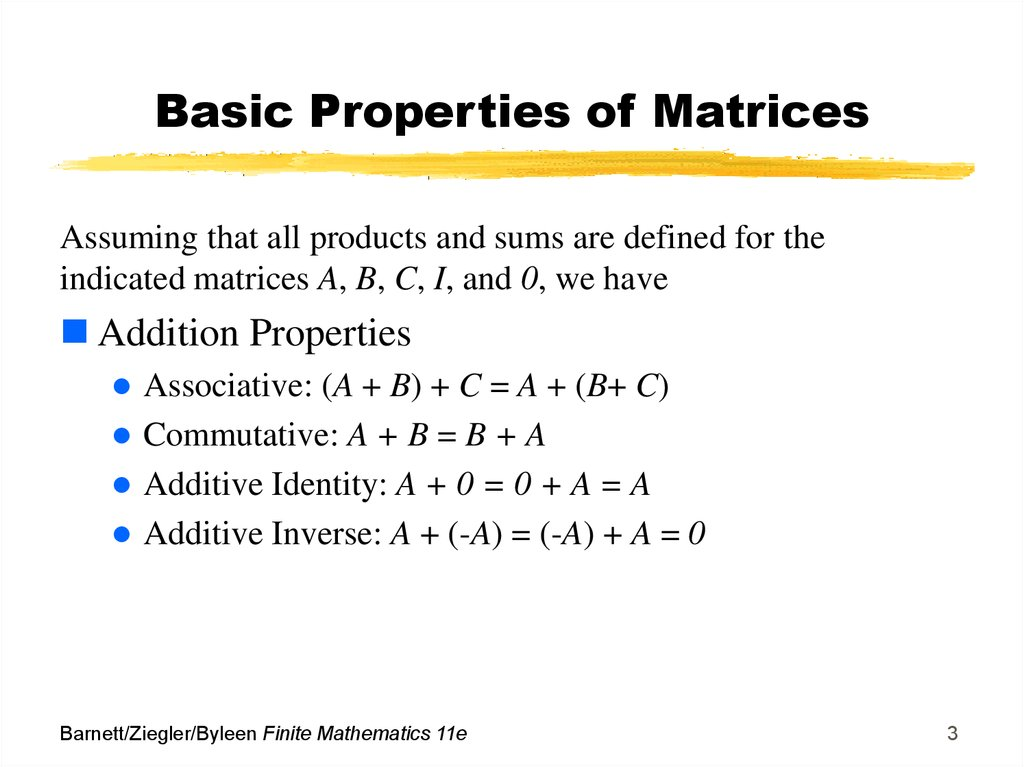# Matrix Equations and Systems of Linear Equations

## 1. Learning Objectives for Section 4.6

Matrix Equations and Systems of
Linear Equations
The student will be able to formulate matrix equations.
The student will be able to use matrix equations to solve linear
systems.
The student will be able to solve applications using matrix
equations.
Barnett/Ziegler/Byleen Finite Mathematics 11e
1

## 2. Matrix Equations

Let’s review one property of solving equations involving real
numbers. Recall
1
b
If ax = b then x = b ,
or
a
a
A similar property of matrices will be used to solve systems of
linear equations.
Many of the basic properties of matrices are similar to the
properties of real numbers, with the exception that matrix
multiplication is not commutative.
Barnett/Ziegler/Byleen Finite Mathematics 11e
2

## 3. Basic Properties of Matrices

indicated matrices A, B, C, I, and 0, we have
Associative: (A + B) + C = A + (B+ C)
Commutative: A + B = B + A
Additive Identity: A + 0 = 0 + A = A
Additive Inverse: A + (-A) = (-A) + A = 0
Barnett/Ziegler/Byleen Finite Mathematics 11e
3

## 4. Basic Properties of Matrices (continued)

Multiplication Properties
Associative Property: A(BC) = (AB)C
Multiplicative identity: AI = IA = A
Multiplicative inverse: If A is a square matrix and A-1
exists, then AA-1 = A-1A = I
Combined Properties
Left distributive: A(B + C) = AB + AC
Right distributive: (B + C)A = BA + CA
Barnett/Ziegler/Byleen Finite Mathematics 11e
4

## 5. Basic Properties of Matrices (continued)

Equality
Addition: If A = B, then A + C = B + C
Left multiplication: If A = B, then CA = CB
Right multiplication: If A = B, then AC = BC
The use of these properties is best illustrated by an example of
solving a matrix equation.
Example: Given an n x n matrix A and an n x p matrix B and a
third matrix denoted by X, we will solve the matrix equation
AX = B for X.
Barnett/Ziegler/Byleen Finite Mathematics 11e
5

## 6. Solving a Matrix Equation

AX B
A
1
AX A
A A X A
1
In X
1
1
1
A B
1
X A B
B
B
Reasons for each step:
1. Given; since A is n x n,
X must by n x p.
2. Multiply on the left by A-1.
3. Associative property of matrices
4. Property of matrix inverses.
5. Property of the identity matrix
6. Solution. Note A-1 is on the left of
B. The order cannot be reversed
because matrix multiplication is
not commutative.
Barnett/Ziegler/Byleen Finite Mathematics 11e
6

## 7. Example

Example: Use matrix inverses to
solve the system
x
y
2 z 1
2x
y
2
x
Barnett/Ziegler/Byleen Finite Mathematics 11e
2 y 2 z 3
7

## 8. Example

Example: Use matrix inverses to
solve the system
Solution:
Write out the matrix of
coefficients A, the matrix X
containing the variables x, y,
and z, and the column matrix
B containing the numbers on
the right hand side of the
equal sign.
Barnett/Ziegler/Byleen Finite Mathematics 11e
x
y
2 z 1
2x
y
2
x
2 y 2 z 3
1 1 2
A 2 1 0
1 2 2
1
x
B 2
X y
3
z
8

## 9. Example (continued)

Form the matrix equation AX = B. Multiply the 3 x 3
matrix A by the 3 x 1 matrix X to verify that this
multiplication produces the 3 x 3 system at the bottom:
1
2
1
1
1
2
2
0
2
x
1
y 2
3
z
x
y
2 z
2x
y
2
x
2 y
2 z 3
Barnett/Ziegler/Byleen Finite Mathematics 11e
1
9

## 10. Example (continued)

If the matrix A-1 exists, then the
solution is determined by
multiplying A-1 by the matrix B.
Since A-1 is 3 x 3 and B is 3 x 1,
the resulting product will have
dimensions 3 x 1 and will store
the values of x, y and z.
A-1 can be determined by the
methods of a previous section
or by using a computer or
calculator. The resulting
equation is shown at the right:
Barnett/Ziegler/Byleen Finite Mathematics 11e
1
X A B
1 1
2 2
X 1 0
3 1
4 4
1
2 1
1 2
1 3
4
10

## 11. Example Solution

The product of A-1 and B is
X A 1 B
1
2
X 1
3
4
1
2
0
1
4
1
2 1
1 2
1 3
4
0
X 2
1
2
Barnett/Ziegler/Byleen Finite Mathematics 11e
The solution can be read off
from the X matrix:
x = 0,
y = 2,
z = -1/2
Written as an ordered triple
of numbers, the solution is
(0, 2, -1/2)
11

## 12. Another Example

Example: Solve the system on the
right using the inverse matrix method.
x 2y z 1
2x y 2z 2
3x y 3z 4
Barnett/Ziegler/Byleen Finite Mathematics 11e
12

## 13. Another Example

Example: Solve the system on the
right using the inverse matrix method.
x 2y z 1
2x y 2z 2
3x y 3z 4
Solution:
The coefficient matrix A is displayed at
the right. The inverse of A does not
exist. (We can determine this by using
a calculator.) We cannot use the inverse
matrix method. Whenever the inverse
of a matrix does not exist, we say that
the matrix is singular.
Barnett/Ziegler/Byleen Finite Mathematics 11e
1 2 1
2 1 2
3 1 3
13

## 14. Cases When Matrix Techniques Do Not Work

There are two cases when inverse methods will not work:
1. If the coefficient matrix is singular
2. If the number of variables is not the same as the number
of equations.
Barnett/Ziegler/Byleen Finite Mathematics 11e
14

## 15. Application

Production scheduling: Labor and material costs for
manufacturing two guitar models are given in the table below:
Suppose that in a given week \$1800 is used for labor and
\$1200 used for materials. How many of each model should be
produced to use exactly each of these allocations?
Guitar model
Labor cost
Material cost
A
\$30
\$20
B
\$40
\$30
Barnett/Ziegler/Byleen Finite Mathematics 11e
15

## 16. Solution

Let x be the number of model
A guitars to produce and y
represent the number of model
B guitars. Then, multiplying
the labor costs for each guitar
by the number of guitars
produced, we have
30x + 40y = 1800
Since the material costs are
\$20 and \$30 for models A and
B respectively, we have
20x + 30y = 1200.
Barnett/Ziegler/Byleen Finite Mathematics 11e
This gives us the system of
linear equations:
30x + 40y = 1800
20x + 30y = 1200
We can write this as a matrix
equation:
30 40 x 1800
20 30 y 1200
16

## 17. Solution (continued)

X A 1 B
30
A
20
40
30
Solution:
Produce 60 model A guitars
and no model B guitars.
0.3 0.4
The inverse of matrix A is
0.2
0.3
x 0.3 0.4 1800 60
y 0.2 0.3 1200 0
Barnett/Ziegler/Byleen Finite Mathematics 11e
17